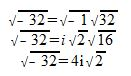# Simplifying Complex Expressions That Contain Square Roots

An error occurred trying to load this video.

Try refreshing the page, or contact customer support.

Coming up next: Radicands and Radical Expressions

### You're on a roll. Keep up the good work!

Replay
Your next lesson will play in 10 seconds
• 0:01 Complex Expressions
• 0:54 The Letter i
• 1:58 Simplifying a Square Root
• 3:40 Simplifying a Complex…
• 5:17 Lesson Summary

Want to watch this again later?

Timeline
Autoplay
Autoplay
Speed

#### Recommended Lessons and Courses for You

Lesson Transcript
Instructor: Yuanxin (Amy) Yang Alcocer

Amy has a master's degree in secondary education and has taught math at a public charter high school.

Watch this video lesson to learn the process of simplifying square roots in complex expressions. Learn how to use the letter i and how to reduce your square roots.

## Complex Expressions

In this video lesson, we will talk about complex expressions. These are the mathematical expressions that include complex numbers, which contain both a real part and an imaginary part. Think of the imaginary part of a mathematical expression as your shadow when you are in the shade. You know you have a shadow, but when you're in the shade, you don't see it. The same is true with a complex expression; you know it has an imaginary part, but you just can't see it on the number line.

Complex numbers look like binomials in that they have two terms. For example, 3 + 4i is a complex number as well as a complex expression. It looks like a binomial with its two terms. See the letter i? That tells you which part is the imaginary part. In this case, 4 is the imaginary part.

## The Letter i

Let's talk about i for a bit. We define i as the square root of -1. So, i squared equals -1. We call the i imaginary because there is no such thing as the square root of a negative number. In the real world, the square root of -1 is undefined. Try taking the square root of -1 on a calculator and you will see that just you can't do it. Just like your shadow is not there when you are in the shade, the same part is true with this imaginary part. However, i is useful in dealing with some complex theoretical problems that you may encounter in your later math studies.

When it comes to simplifying our complex expressions, the letter i comes in handy when we need to deal with negative square roots. Because the i is defined as the square root of -1, we can use it to simplify any square root. For example, while the square root of -9 is not valid in the real world, by using the letter i we can simplify it to 3i in a complex expression.

## Simplifying a Square Root

How did we simplify the square root of -9 to 3i? We split the square root of -9 into two square roots multiplied with each other. We have the square root of -1 times the square root of 9. We know that the square root of 9 is 3, and from our definition of i we have the square root of -1 as i. So, we end up with the square root of -9 being 3i.

Let's look at simplifying another square root: the square root of -32. How do we do that? We first split this square root into the square root of -1 times the square root of 32. Then we can substitute an i for the square root of -1. Now, we can work on simplifying the square root of 32. We can split the square root of 32 into the square root of 2 times the square root of 16. We can split any square root into any two numbers that when multiplied together gives us our original number. We should know the square root of one of those numbers.

In our case, we chose 2 and 16 because 2 * 16 = 32, and because we know the square root of 16. So, we have the square root of 2 times the square root of 16 equals the square root of 32. We know the square root of 16 is 4, so we can substitute a 4 for the square root of 16. The square root of 2 we can't do anything about so we leave it as that. After simplifying, we have the square root of -32 equals 4i times the square root of 2.To unlock this lesson you must be a Study.com Member.

### Register to view this lesson

Are you a student or a teacher?

### Unlock Your Education

#### See for yourself why 30 million people use Study.com

##### Become a Study.com member and start learning now.
Back
What teachers are saying about Study.com

### Earning College Credit

Did you know… We have over 160 college courses that prepare you to earn credit by exam that is accepted by over 1,500 colleges and universities. You can test out of the first two years of college and save thousands off your degree. Anyone can earn credit-by-exam regardless of age or education level.